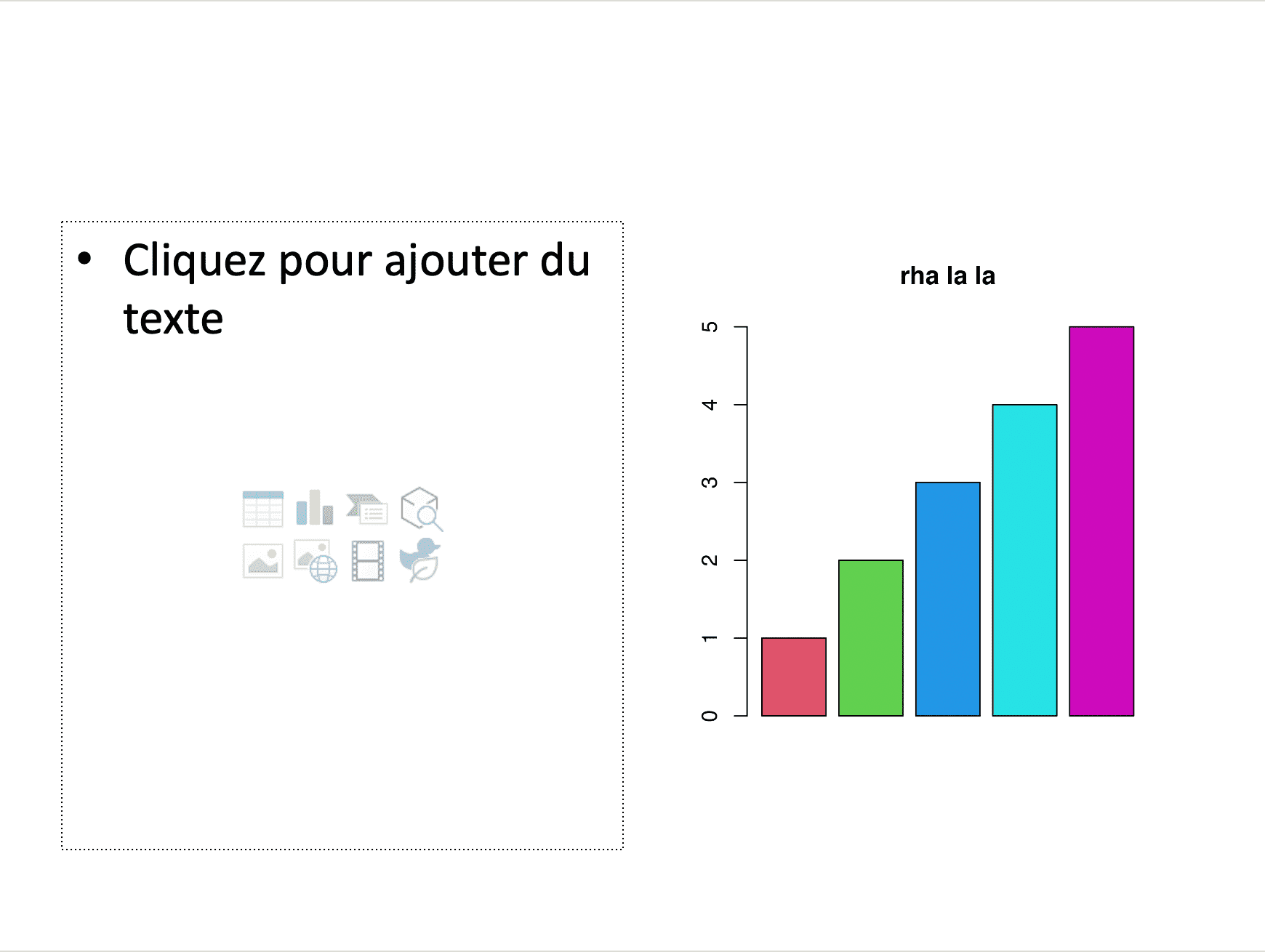# Chapter 8 Paragraphs with chunk objects

## 8.1 Chunk objects

These objects are pieces, text or images that can be arranged to form a paragraph.

For PowerPoint, only the `ftext’ function can be used in the composition of a paragraph1.

For Word, a wider palette is available:

• `ftext()`: formatted text (with a `fp_text` class object)
• `external_img()`: image
• `run_autonum()`: Create a self-numbered piece, i.e. the representation of a sequence, each element will be numbered. These sequences can also be bookmarked and used later for cross-referencing.
• `run_columnbreak()`: column break in section
• `run_linebreak()`: soft return
• `run_pagebreak()`: page break
• `run_reference()`: reference
• `run_word_field()`: Word computed field
• `run_bookmark()`: add a bookmark
• `run_footnote()`: add a footnote

## 8.2 Paragraphs made with `fpar`

This function allows you to create paragraphs made of chunks as described upper. The result can be inserted in a Word document and in a PowerPoint document.

``````red_font <- fp_text(font.size = 60, color = "red", bold = TRUE)
pink_font <- update(red_font, color = "gray", italic = TRUE)

a_par <- fpar(
ftext("hello", red_font),
" ",
ftext("world", pink_font),
fp_p = fp_par(text.align = "center", padding = 5)
)``````

hello world

``````fileout <- tempfile(fileext = ".pptx")
doc_1 <- add_slide(doc_1, layout = "Title and Content", master = "Office Theme")
doc_1 <- ph_with(
x = doc_1, value = a_par,
location = ph_location_type(type = "body"))
print(doc_1, target = "reports/example_fpar_1.pptx")````````````img.file <- file.path( R.home("doc"), "html", "logo.jpg" )

normal_font <- fp_text(font.size = 20)
bold_font <- update(normal_font, font.size = 20)
bold_red_font <- update(bold_font, color = "red")

a_par <- fpar(
ftext("Hello World, ", prop = normal_font ),
ftext("how ", prop = bold_font ),
external_img(src = img.file, height = 1.06/2, width = 1.39/2),
ftext(" you?", prop = bold_red_font ),
fp_p = fp_par(text.align = "center", padding = 5))``````

Hello World, how you?

``````read_docx() %>%
print(target = "reports/example_fpar_2.docx")``````## 8.3 Creating paragraphs with Word computed fields

One of the nice features of the package is to allow the insertion of fields calculated by Word. Currently, you can insert references, auto-numbered sequences, page breaks, rows, columns and any calculated field as long as you know the code to use.

• `run_autonum()`: Create a self-numbered piece, i.e. the representation of a sequence, each element will be numbered. These sequences can also be bookmarked and used later for cross-referencing.
• `run_columnbreak()`: column break in section
• `run_linebreak()`: soft return
• `run_pagebreak()`: page break
• `run_reference()`: reference
• `run_word_field()`: Word computed Field, user can add any Word computed field
``````fp_t <- fp_text(font.size = 12, bold = TRUE)
an_fpar_1 <- fpar("let's add an auto-number: ",
run_autonum(seq_id = "seqid", pre_label = "sequence ", bkm = "seq1"),
ftext("and some blah blah! ", fp_t),
run_seqfield("DATE \\@ \"dddd, MMMM d\"", prop = fp_t)
)
an_fpar_2 <- fpar("let's add an auto-number: ",
run_autonum(seq_id = "seqid", pre_label = "sequence ", bkm = "seq2"),
ftext("and some blah blah! ", fp_t)
)
an_fpar_3 <- fpar("let's add an auto-number: ",
run_autonum(seq_id = "seqid", pre_label = "sequence ", bkm = "seq3"),
ftext("and some blah blah! ", fp_t)
)

print(target = "reports/example_fpar_3.docx")``````## 8.4 List of blocks

Function `block_list` enable to build a list of blocks of paragraphs. The function is to be used when adding formatted paragraphs into a Word document or a PowerPoint presentation.

``````b_par <- fpar(
ftext("How are you tody? ", prop = normal_font ),
ftext("- not too bad", prop = bold_font ),
fp_p = fp_par(text.align = "right", padding.top = 10))``````

Hello World, how you?

How are you tody? - not too bad

Here is an example with Word:

``````read_docx() %>%
print(target = "reports/example_block_list_1.docx")``````The example below show how to use it with `ph_with` method for PowerPoint production. These objects can also in this context be formatted as unordered lists, see Multiple paragraphs.

``````fpt_blue_bold <- fp_text(color = "#006699", bold = TRUE, font.size = 30)
fpt_red_italic <- fp_text(color = "#C32900", italic = TRUE, font.size = 30)
value <- block_list(
fpar(ftext("hello world", fpt_blue_bold)),
fpar(ftext("hello", fpt_blue_bold), " ",
ftext("world", fpt_red_italic)),
fpar(
ftext("blah blah blah", fpt_red_italic)))

doc <- ph_with(doc, value, location = ph_location_type(type = "body"))
print(doc, target = "reports/example_block_list_2.pptx")``````## 8.5 R plot

`plot_instr`is a simple wrapper to capture plot instructions that will be executed and copied in a document. The function enable usage of any R plot with argument code. Wrap your code between curly bracket if more than a single expression.

The function does not handle multi plot instructions.

``````anyplot <- plot_instr(code = {
barplot(1:5, col = 2:6)
title(main = "rha la la")
})

doc <- ph_with(
doc, anyplot,
location = ph_location_fullsize(),
bg = "#00000066", pointsize = 12)
print(doc, target = "reports/example_anyplot_1.pptx")````````````doc <- read_docx()
doc <- body_add(doc, anyplot, width = 5, height = 4)
print(doc, target = "reports/example_anyplot_2.docx")``````## 8.6 Empty content

The object returned by the `empty_content` function allows you to add empty content to a slide. This comes in handy when presentation elements are added or updated, but you want to leave the user a place where they can write things manually.

``````doc <- read_pptx()
doc <- add_slide(doc, layout = "Two Content")
doc <- ph_with(x = doc, value = empty_content(), location = ph_location_left() )
doc <- ph_with(x = doc, value = anyplot, location = ph_location_right() )
print(doc, target = "reports/example_empty_content.pptx" )``````## 8.7 Simple list for PowerPoint

`unordered_list()` enable the creation of an unordered list of text for PowerPoint presentations. Each text is associated with a hierarchy level.

Text formatting of paragraphs can be customized with the argument `style`. If you enter an object of type `fp_text`, the formatting will apply to all paragraphs. If you enter a list of `fp_text` objects (one for each paragraph) then each paragraph will be formatted with its associated style. By default, the value is set to `NULL` which is interpreted as an indication not to apply formatting.

``````ul <- unordered_list(
level_list = c(1, 2, 3),
str_list = c("Level1", "Level2", "Level1"),
style = list(
fp_text(color = "#C32900", font.size = 0),
fp_text(color = "#006699", font.size = 0),
fp_text(color = "#f2af00", font.size = 0)
))